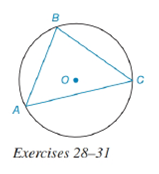Chapter 6.4, Problem 28E### Elementary Geometry for College St...

6th Edition
Daniel C. Alexander + 1 other
ISBN: 9781285195698

#### Solutions

Chapter
Section### Elementary Geometry for College St...

6th Edition
Daniel C. Alexander + 1 other
ISBN: 9781285195698
Textbook Problem
1 views

# Given that m A C ⌢ : m B C ⌢ : m A B ⌢ = 4 : 3 : 2 in circle O :a) Which arc is largest?b) Which chord is longest?To determine

(a)

To find:

The largest arc.

Explanation

Given:

mAC:mBC:mAB=4:3:2 in circle O. The figure given below

Theorem Used:

In a circle (or in congruent circles) containing two unequal arcs, the larger arc corresponds to the larger central angle.

Calculation:

Since, mAC:mBC:mAB=4:3:2 and the sum of the arcs is 360°, we get

mAC+mBC+mAB=360°

or 4x+3x+2x=360°

To determine

(b)

To find:

The longest chord.

### Still sussing out bartleby?

Check out a sample textbook solution.

See a sample solution

#### The Solution to Your Study Problems

Bartleby provides explanations to thousands of textbook problems written by our experts, many with advanced degrees!

Get Started